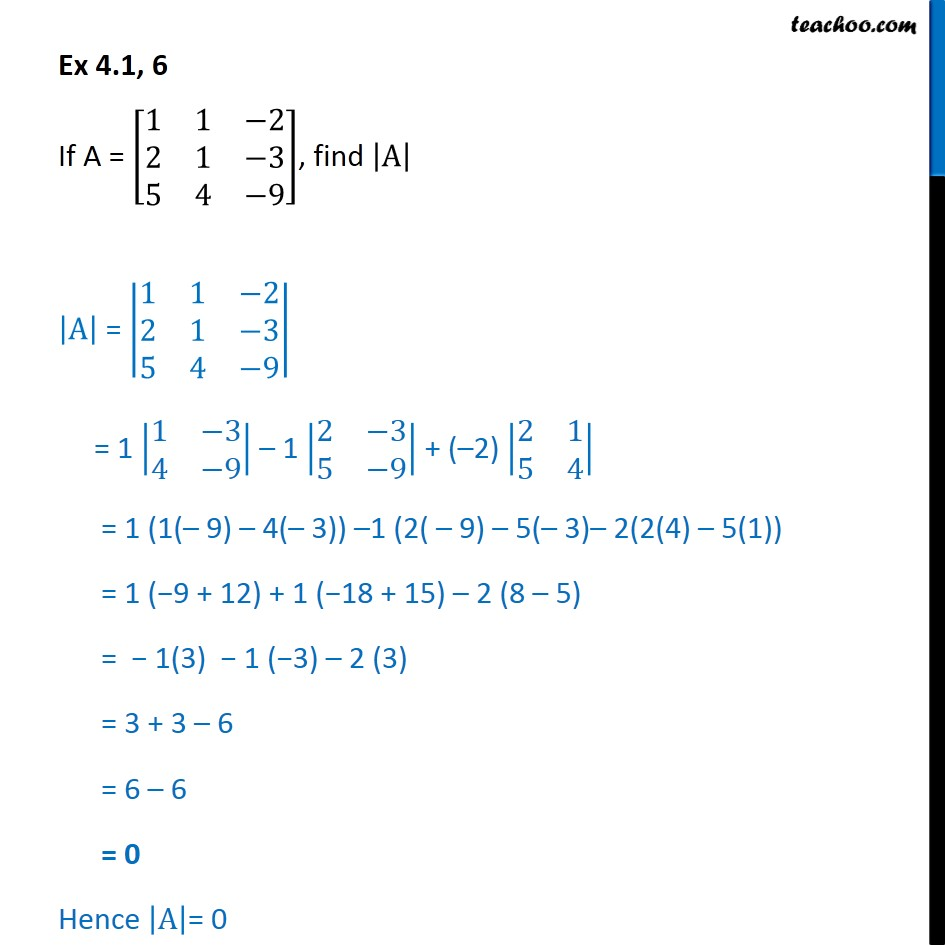1. Chapter 4 Class 12 Determinants
2. Serial order wise
3. Ex 4.1

Transcript

Ex 4.1, 6 If A = 1 1 2 2 1 3 5 4 9 , find A A = 1 1 2 2 1 3 5 4 9 = 1 1 3 4 9 1 2 3 5 9 + ( 2) 2 1 5 4 = 1 (1( 9) 4( 3)) 1 (2( 9) 5( 3) 2(2(4) 5(1)) = 1 ( 9 + 12) + 1 ( 18 + 15) 2 (8 5) = 1(3) 1 ( 3) 2 (3) = 3 + 3 6 = 6 6 = 0 Hence A = 0

Ex 4.1